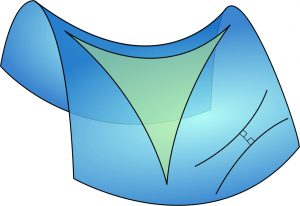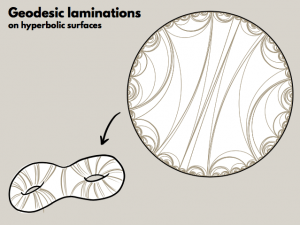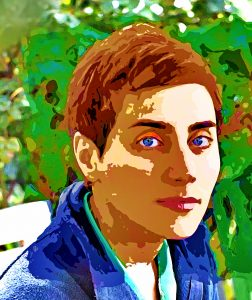# The mathematics of Maryam Mirzakhani

We take a proper look at her mathematical accomplishmentsMaryam Mirzakhani, the first woman Fields medallist and an explorer of abstract surfaces, left us in the prime of her life. Rightly, the world press mourned her passing, but what I hope to do here is to write about the beautiful and difficult mathematics she loved working on. As a pure mathematician, she was usually driven by in-depth understanding of the different complex structures on abstract surfaces, rather than the search for application. Nevertheless, her work has been used in solving real life problems. But what I personally find fascinating about her is the courage and creativity she had in attempting and solving long standing problems and the variety of areas within mathematics she worked on; from complex geometry and topology to dynamical systems. Here is a more intuitive exposition of some of her achievements.

## SurfacesA tasty surface. Image: Descubra Sorocaba, CC BY 2.0

I am sure that if I asked you to give me an example of a surface you would be able to do so straight away. You might say the surface of the Earth is obviously one, and you would be right. However, defining what we mean by a surface mathematically is a little bit trickier. Let’s give it a go. A geometrical object is called a surface if, when we zoom in very closely at the points on the shape, we can see overlapping patches of the plane. If we were to use mathematical language we would say that a surface is locally homeomorphic to the plane.A genus 1 surface

You might not find this definition particularly helpful so let us consider a few more examples. Oranges, tomatoes, apples and, for more delicious alternatives, cakes, cupcakes, ring doughnuts and pretzels are all surfaces. Well, almost! In order for them to be surfaces we need to picture them hollow (or like a balloon), rather than solid. If we consider those objects geometrically, meaning that we differentiate between different angles and size lengths, we notice that there are infinitely many of them. This is why we consider them topologically. Using continuous deformations we can turn almost all of our examples into a sphere, except the ones that have holes in them. They are considered to be in a class of their own. Thus we can classify the surfaces up to deformations (topological equivalence) by the number of holes, which we call the genus. We can see that the sphere has genus 0, the torus (ring doughnut) genus 1 and the 3-fold torus (pretzel) genus 3, thus these are all inequivalent surfaces.

Mirzakhani’s work was on Riemann surfaces. To turn a surface into a Riemann surface we need to give it additional geometric structure. For example, we can give the surface geometric structure that allows us to measure angles, lengths and area. An example of such geometry is hyperbolic geometry. It is the first example of non-Euclidean geometry; the only way it differentiates from Euclidean geometry is that given a line $\ell$ and a point $P$, that is not on the line, we can draw at least 2 distinct lines through $P$ that are parallel to $\ell$.Parallel lines and a triangle on a hyperbolic surface

One peculiar consequence of this new axiom is that rectangles do not exist in hyperbolic geometry. Moreover, the angle sum for a triangle is always less than $\pi$. Mirzakhani’s early work was on hyperbolic surfaces, which are Riemann surfaces with hyperbolic structure. The problem with hyperbolic surfaces is that we cannot really visualise them, because the hyperbolic structure on the Riemann surface can’t be embedded in $\mathbb{R}^3$. However, we can try and describe roughly how you put the structure on the surface. Imagine our surface is made out of rubber and we can bend it and fold it in all dimensions. Now we add the hyperbolic structure, but for that we need, according to John Nash, 17 dimensions. If we next dip it in cement it becomes solid, and we can no longer stuff it into 3 dimensions, hence we can no longer visualise it completely.

On these surfaces, Mirzakhani studied special objects called closed geodesics. Roughly speaking, a geodesic is a generalisation of the notion of a straight line that we have on the Euclidean plane. We can define a geodesic more rigorously as a path between two points on the surface, whose length cannot be shortened by deforming it. For example, on the sphere, the geodesics are called great circles. These are simply the intersections of a plane going through the origin and the sphere itself.A sphere sliced in half along a great circle

A closed geodesic is a geodesic that starts and ends at the same point. The simplest example of a closed geodesic is a circle.We also allow intersections, that is, geodesics that look, for example, like a figure-of-eight and are much more complicated. Using the hyperbolic structure on the Riemann surface, we can compute the lengths of these closed geodesics. A natural question we can ask is how many such closed geodesics are there on any hyperbolic surface of length $\leq L$? The answer was established in the 1940s by Delsarte, Huber and Selberg and it was named the prime number theorem for hyperbolic surfaces, because of the resemblance to the prime number theorem. That is, the number of closed geodesics, denoted by $\pi$, satisfies\begin{align*} \pi(X, L)\sim \mathrm{e}^L/L,\end{align*}as $L\rightarrow \infty$. Roughly speaking, the number of closed geodesics on a hyperbolic surface $X$ of length $\leq L$ gets closer to $\mathrm{e}^{L}/L$ as $L$ becomes very big. We can see that their number grows exponentially, meaning very quickly, but more importantly we also see that the formula does not depend on the surface we are on.

The next question to consider is what would happen if we no longer allow our geodesics to intersect themselves? Would our formula change much? Will the growth rate be significantly different? That is, we wish to compute the number of simple closed geodesics (simple meaning no intersections are allowed) on a hyperbolic surface $X$ of length $\leq L$, denoted $\sigma(X, L)$. In 2004, Mirzakhani proved, as part of her PhD thesis, that \begin{align*} \sigma(X,L)\sim C_{X} L^{6g-6},\quad\text{as }L\to\infty,\end{align*}where $g$ denotes the genus of the surface $X$, and $C_{X}$ is some constant dependent on the geometry (hyperbolic structure) of the surface. It is important to make clear that surfaces of a given genus can be given many different hyperbolic structures. As a consequence the number grows much slower (polynomially) but it also depends on the surface we are on. The surface may be the same, but the different structure implies that we would have different geodesics and their lengths would also be different. Whilst she was computing $\sigma(X,L)$, she discovered formulae for the frequencies of different topological types of simple closed curves on $X$. The formulae are a bit too complicated to explain here, but let us consider an example: suppose $X$ is a surface of genus 2; there is a probability of 1/7 that a random simple closed geodesic will cut the surface into two genus 1 pieces. How cool is that?!Flight paths follow geodesics on the Earth’s surface

Even though these results are for a given hyperbolic structure, Mirzakhani proved it by considering all structures at the same time. We know that we can continuously deform surfaces of the same genus $g$ and they will be topologically the same, however geometrically they may be different. These deformations depend on $6g-6$ parameters, which was known to Riemann. We call these parameters moduli and we can consider their space, the so-called moduli space of all hyperbolic structures on a given topological surface. By definition, a moduli space is a space of solutions of geometric classification problems up to deformations. This is a bit abstract, so let us illustrate it with a simple example. Suppose our geometric classification problem is to classify the circles in Euclidean space up to equivalence. We would say that two circles are equivalent if they have the same radius, no matter where their centre lies. That is, our modulus (parameter) is the radius $r$ of the circle, and we know that $r\in\mathbb{R}^{+}$. Hence the moduli space will be the positive real numbers. So what can we do with these new spaces? Greg McShane observed that you can add a new structure; a so-called symplectic structure which, roughly speaking, allows us to measure volumes on moduli spaces. Mirzakhani found a connection between volumes on moduli spaces and the number of simple closed geodesics on one surface. She computed some specific volumes on moduli spaces and her celebrated result followed.

## Dynamical systems“Pot as many balls as you can.” Image: Curtis Perry, CC BY-NC-SA 2.0

In recent years, Mirzakhani focused her attention on dynamical systems on moduli spaces. A dynamical system is simply a system that evolves with time. Originally, dynamical systems arose in physics by looking at the movements of particles in a chamber or planets in the solar system. It was observed that these large systems are similar to smaller ones, and by studying toy models we might shed some light on the actual physical dynamical systems.

One such toy model is the dynamical system of billiard balls on a polygonal table (not necessarily rectangular). Bear in mind that in this version of billiards we only use one ball and it can travel forever on a path as long as it doesn’t reach a corner. The billiard balls will take the shortest paths, thus they travel via geodesics, and this is where Mirzakhani’s research come into play. As we know by now, she studied surfaces rather than polygons, but if you orient the edges of the table in pairs and glue them together then you can turn it into a surface.

Even though billiard dynamics might seem simple, there are difficult problems that are still unsolved. One might ask if there are any periodic billiard paths, and if so, would the answer change if we change the shape of the table $T$? This problem has been solved: it is known that there is always at least one periodic billiard path for a rational polygonal table (by a rational polygon, we mean a polygon whose angles are rational multiples of $\pi$). But what if we now ask what is the number of such periodic billiard paths of length $\leq L$ on a table $T$, denoted by $N(T, L)$? It is conjectured that the following asymptotic formula holds.

\begin{align*} N(T, L)\sim \frac{C_T L^2}{\pi \text{ Area}(T)},\end{align*}where $C_T$ is some constant depending on the table. Alongside Alex Eskin and Amir Mohammadi, Mirzakhani made some progresstowards this result. They showed that $\lim_{n\to\infty}N(T,L)/L^2$ exists and is non-zero. Mirzakhani’s work unfortunately does not provide a solution, however she brought progress by showing that this number satisfies the following asymptotic formulaMoreover, she showed that for the asymptotic formula to even exist in the form above, there exist only countably many numbers $C_T$. Recently, Mirzakhani and Eskin’s work on billiard paths was applied to the sight lines of security guards in complexes of mirrored rooms.Aaron Fenyes

Another example of her impact on dynamical systems is her work on Thurston’s earthquake flow. Suppose we have a Riemann surface $X$ of genus $g$, a simple closed geodesic $\gamma$ on $X$ and a real number $t$. Then we obtain a new Riemann surface $X_t= \text{tw}_{\gamma}^t(X)$ by cutting $X$ along $\gamma$, twisting it to the right by $t$ and re-gluing. Then we can define the flow at time $t$ to be

\begin{align*} \text{tw}^{t}(\lambda, X\hspace{0.3mm})=(\lambda, \text{tw}_{\lambda}^t(X\hspace{0.3mm}))\end{align*}

where $\lambda$ is geodesic lamination. The definition of geodesic lamination is quite technical, so in this article we can simply think of it as a disjoint collection of simple geodesics on $X$. Intuitively, we have a dynamical system like the movement of planets in time $t$, but in our case the objects that move with time are moduli spaces.

We get some sort of periodicity, because if $\gamma$ has length $L$, then $X_{L+t}=X_t$. Mirzakhani showed something truly remarkable: The earthquake flow is ergodic.
This means that if we follow the laminations along we would be very close to any point on the surface with probability 1. This came as a surprise, because until then there was not a single known example showing that the earthquake paths are dense.